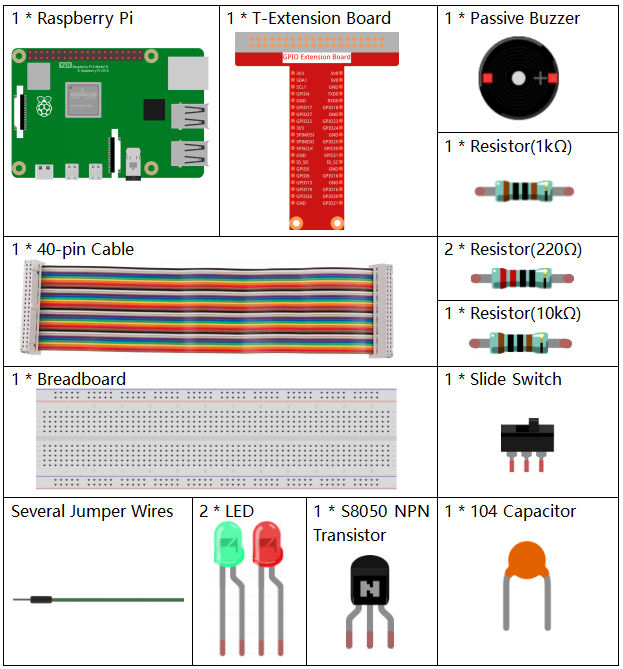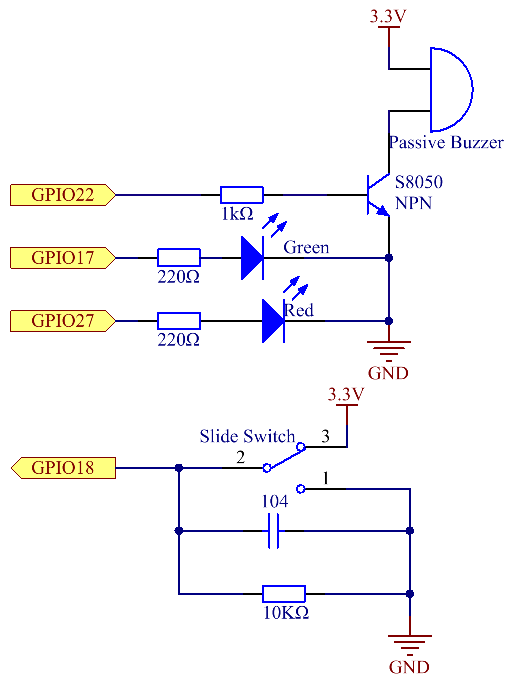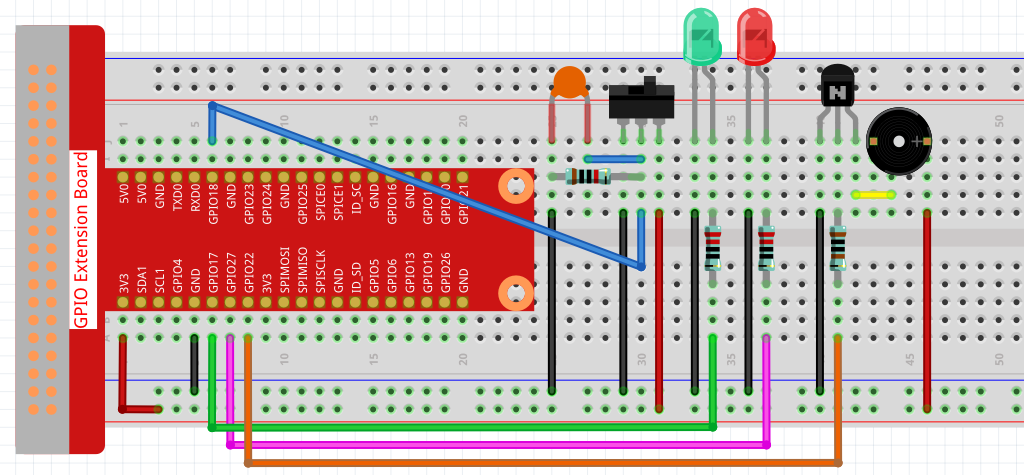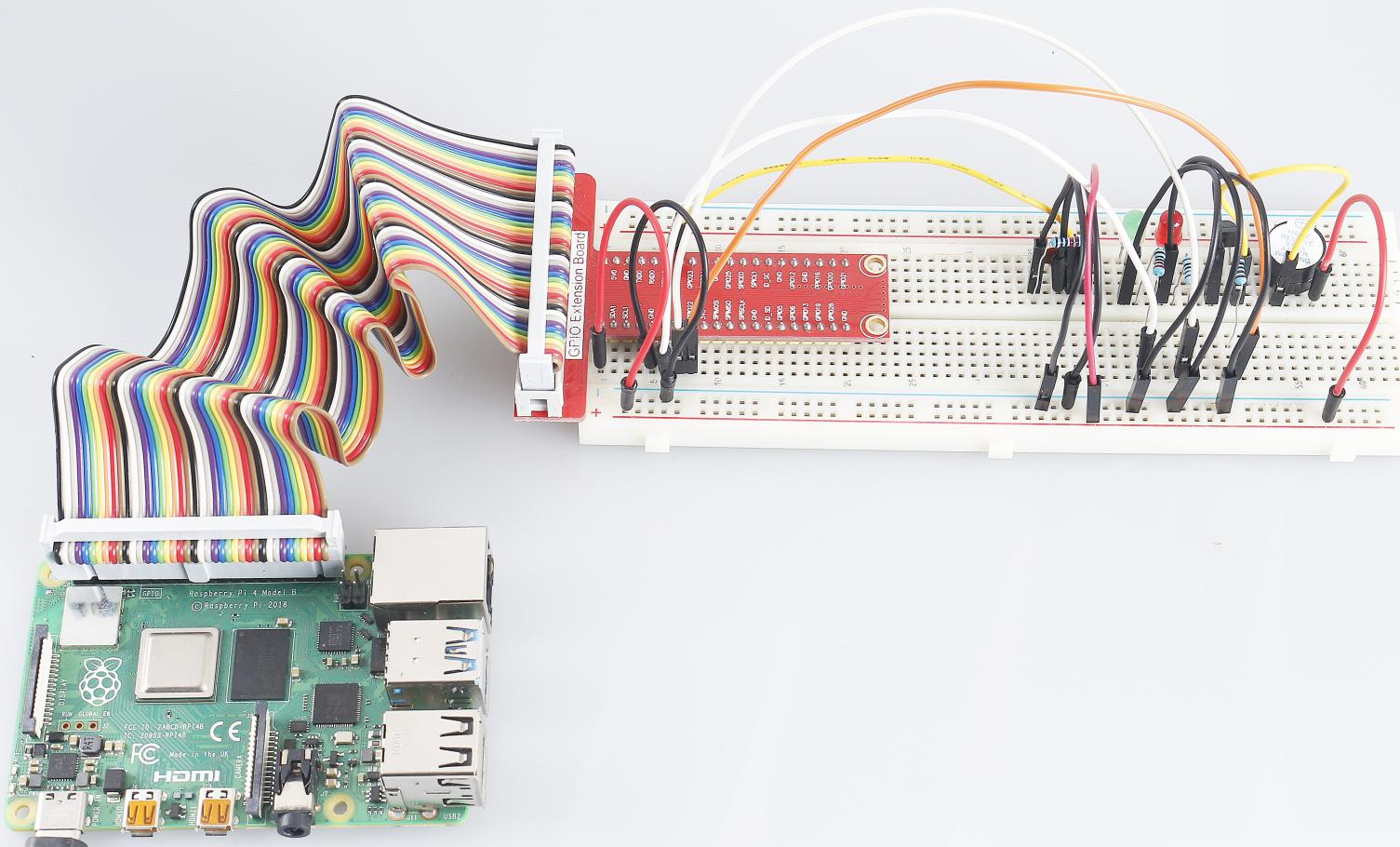# 4.1.15 Alarm Bell¶

## Introduction¶

In this project, we will make a manual alarm device. You can replace the toggle switch with a thermistor or a photosensitive sensor to make a temperature alarm or a light alarm.

## Required Components¶

In this project, we need the following components.Name

ITEMS IN THIS KIT

Raphael Kit

337

Raphael Kit

COMPONENT INTRODUCTION

GPIO Extension Board

Jumper Wires

Resistor

LED

Buzzer

Slide Switch

Transistor

Capacitor

## Schematic Diagram¶

 T-Board Name physical wiringPi BCM GPIO17 Pin 11 0 17 GPIO18 Pin 12 1 18 GPIO27 Pin 13 2 27 GPIO22 Pin 15 3 22## Experimental Procedures¶

Step 1: Build the circuit.Step 2: Change directory.

```cd ~/raphael-kit/python/
```

Step 3: Run.

```sudo python3 4.1.15_AlarmBell.py
```

After the program starts, the toggle switch will be toggled to the right, and the buzzer will give out alarm sounds. At the same time, the red and green LEDs will flash at a certain frequency.

Code

Note

You can Modify/Reset/Copy/Run/Stop the code below. But before that, you need to go to source code path like `raphael-kit/python`.

```#!/usr/bin/env python3

import RPi.GPIO as GPIO
import time

BeepPin=22
ALedPin=17
BLedPin=27
switchPin=18

Buzz=0
flag =0
note=150
pitch=20

def setup():
GPIO.setmode(GPIO.BCM)
GPIO.setup(BeepPin, GPIO.OUT)
GPIO.setup(ALedPin,GPIO.OUT,initial=GPIO.LOW)
GPIO.setup(BLedPin,GPIO.OUT,initial=GPIO.LOW)
GPIO.setup(switchPin,GPIO.IN)
global Buzz
Buzz=GPIO.PWM(BeepPin,note)

def ledWork():
while flag:
GPIO.output(ALedPin,GPIO.HIGH)
time.sleep(0.5)
GPIO.output(ALedPin,GPIO.LOW)
GPIO.output(BLedPin,GPIO.HIGH)
time.sleep(0.5)
GPIO.output(BLedPin,GPIO.LOW)

def buzzerWork():
global pitch
global note
while flag:
if note >= 800 or note <=130:
pitch = -pitch
note = note + pitch
Buzz.ChangeFrequency(note)
time.sleep(0.01)

def on():
global flag
flag = 1
Buzz.start(50)
tBuzz.start()
tLed.start()

def off():
global flag
flag = 0
Buzz.stop()
GPIO.output(ALedPin,GPIO.LOW)
GPIO.output(BLedPin,GPIO.LOW)

def main():
lastState=0
while True:
currentState =GPIO.input(switchPin)
if currentState == 1 and lastState == 0:
on()
elif currentState == 0 and lastState == 1:
off()
lastState=currentState

def destroy():
off()
GPIO.cleanup()

if __name__ == '__main__':
setup()
try:
main()
except KeyboardInterrupt:
destroy()
```

Code Explanation

```import threading
```

Here, we import the `Threading` module and it allows you to do multiple things at once, while normal programs can only execute code from top to bottom. With `Threading` modules, the LED and the buzzer can work separately.

```def ledWork():
while flag:
GPIO.output(ALedPin,GPIO.HIGH)
time.sleep(0.5)
GPIO.output(ALedPin,GPIO.LOW)
GPIO.output(BLedPin,GPIO.HIGH)
time.sleep(0.5)
GPIO.output(BLedPin,GPIO.LOW)
```

The function `ledWork()` helps to set the working state of these 2 LEDs: it keeps the green LED lighting up for 0.5s and then turns off; similarly, keeps the red LED lighting up for 0.5s and then turns off.

```def buzzerWork():
global pitch
global note
while flag:
if note >= 800 or note <=130:
pitch = -pitch
note = note + pitch
Buzz.ChangeFrequency(note)
time.sleep(0.01)
```

The function `buzzWork()` is used to set the working state of the buzzer. Here we set the frequency as between 130 and 800, to accumulate or decay at an interval of 20.

```def on():
global flag
flag = 1
Buzz.start(50)
tBuzz.start()
tLed.start()
```

In the function `on()` :

1. Define the mark “flag=1”, indicating the ending of the control thread.

2. Start the Buzz, and set the duty cycle to 50%.

3. Create 2 separate threads so that the LED and the buzzer can work at the same time.

`threading.Thread()` function is used to create the thread and its prototype is as follows:

`class threading.Thread(group=None, target=None, name=None, args=(), kwargs={}, *, daemon=None)`

Among the construction methods, the principal parameter is `target`, we need to assign a callable object (here are the functions `ledWork` and `BuzzWork`) to `target`.

Next `start()` is called to start the thread object, ex., `tBuzz.start()` is used to start the newly installed `tBuzz` thread.

```def off():
global flag
flag = 0
Buzz.stop()
GPIO.output(ALedPin,GPIO.LOW)
GPIO.output(BLedPin,GPIO.LOW)
```

The function `Off()` defines “flag=0” so as to exit the threads ledWork and BuzzWork and then turn off the buzzer and the LED.

```def main():
lastState=0
while True:
currentState =GPIO.input(switchPin)
if currentState == 1 and lastState == 0:
on()
elif currentState == 0 and lastState == 1:
off()
lastState=currentState
```

`Main()` contains the whole process of the program: firstly read the value of the slide switch; if the toggle switch is toggled to the right (the reading is 1), the function `on()` is called, the buzzer is driven to emit sounds and the the red and the green LEDs blink. Otherwise, the buzzer and the LED don’t work.

## Phenomenon Picture¶